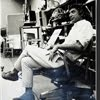## Eugene Ray SDSU 1970'sEugene Ray 1970's. photo credit: Tom Davis

## Saturday, September 27, 2014

### TENSILE STRUCTURES / nature our best guide

>(resistent to hurricanes<
>>and earth quakes)< !
+IS TENSILE & RESILIENT+
^^^^^^^^^^^^^^^^^^^^^

+LINDA MOULTON HOWE+
>(earth files & earth files . com)<
^^^^^^^^^^^^^^^^^^
>is like our profound new research friend<
+STACE TUSSEL-COLLIGAN+
>(inter-intelligence-communications.com)<
^^^^^^^^^^^^^^^^^^^^^^
+IMPRESSIVE RESEARCHERS+
>of ufo and related phenomena like<
>crop circles and cattle mutiliation<
^^^^^^^^^^^^^^^^^^^^^^
+NON-BELIEVERS+
>should listen to a cattle man on "coast to<
+(SEE STACE'S IMPRESSIVE+
+VIDEO DOCUMENTARIES)+
>his cattle in the middle of a long night<
>"not of this world", (his explanation)<
^^^^^^^^^^^^^^^^^^^^^^^
>this 1969 version of the whole earth catalog was<
>a great reference for early sdsu environ design<
+(ENVIRON DESIGN+
+>>PRIMARY REF)+
>classes that i taught w/ biotronics in mind<
>(stewart brand's book won pulitzer prize)<
^^^^^^^^^^^^^^^^^^^^^^^^^^^^
>you some idea of the organic systems side w/<
+(BIOMORPHIC BONE+
+NATURAL DESIGN)+
>exellent reference to tensile natural structure<
>cosmic consciousness was the driving force<
^^^^^^^^^^^^^^^^^^^^^^^^^^^

>two different uses of tensile structure in my<
>oeuvre from tensile fiber optic cable (top)<
+(TENSILE IS LIGHT WT+
+>THUS ECONOMICAL)+
>to bent structural frame for bungalows<
^^^^^^^^^^^^^^^^^^^^^^^^^^^
>this house near new orleans in framing stage<
>reveals a light, open, tensile structure that<
+>>WILTY RESIDENCE+
>was economical and assembled quickly<
>(built 2 mos, w/ two young carpenters)<
^^^^^^^^^^^^^^^^^^^^^^^^^^>i consider this tensile structure design the key<
>design for future generations to my oeuvre of<
+HEALTHY ECONOMICAL)+
>radiant biotronic architecture, good for health<
>(this concept radiant with conoidal energy)<
^^^^^^^^^^^^^^^^^^^^^^^^^^
>my tensile structure conoid for the san diego<
>native american community combines the<
+(CONOID IS SUPERIOR+
>historical "teepee" with fiber optic cables<
>(light weight structure earns economy)<
^^^^^^^^^^^^^^^^^^^^^^^^^^^^
>there is no doubt that monocoque shells are<
>the strongest enclosure known for human<
+(NATURE IS OUR+
+>>BEST GUIDE)+
>habitation, (ferro-cem is economy tech)<
^^^^^^^^^^^^^^^^^^^^^^^
>tensile worm hole, time travel technology<
>may yet save from earth's negative ways<
+(FOR MY OLD FRIEND+
>(be aware of much progress in this)<
^^^^^^^^^^^^^^^^^^^^^^^^^^^^
>cosmic consciousness is essential to radiant<
>architecture and the research of dedicated<
+(TRANSCENDENTAL+
+MANIFESTATIONS)+ !
>scholars like stace tussel colligan and<
>linda moulton howe very important<
^^^^^^^^^^^^^^^^^^^^^^^^

>tensile structure was essential to my sdsu class<
>"synergetic environments", two different<
+(TENSILE SYNERGY @ +
+70s ENVIRON DESIGN)+
>designs we see from my 1970s archive<
>(cosmic consciousness a strong factor)<
^^^^^^^^^^^^^^^^^^^^^^^^
>robert lazar is just one of many talented physics<
>genius whistle blowers who worked for the<
+ROSWELL" BY CORSO)+
>govt on UFO back engineering and have<
>told their inside experience to the world<
^^^^^^^^^^^^^^^^^^^^^^^^^
+DEFENSE MINISTER, CREDITS the+
>revealing books/video)<
+CORSO BOOK for waking him up to+
+THE TRUTHS OF UFO HISTORY+ !
^^^^^^^^^^^^^^^^^^^^^^^^^^
eugene ray, mfa, architect
professor emeritus, sdsu

1.2.